SimpleITK conventions: SimpleITK indexes are zero based, except for the slicing operator which conforms with R conventions and is one based. Points are represented by vector-like data types: vector, array, list. Matrices are represented by vector-like data types in row major order. Initializing the DisplacementFieldTransform using an image requires that the image's pixel type be sitk.sitkVectorFloat64 Initializing the DisplacementFieldTransform using an image will "clear out" your image (your alias to the image will point to an empty, zero sized, image).

# SimpleITK Transformation Types¶

This notebook introduces the transformation types supported by SimpleITK and illustrates how to "promote" transformations from a lower to higher parameter space (e.g. 3D translation to 3D rigid).

 TranslationTransform 2D or 3D, translation VersorTransform 3D, rotation represented by a versor VersorRigid3DTransform 3D, rigid transformation with rotation represented by a versor Euler2DTransform 2D, rigid transformation with rotation represented by a Euler angle Euler3DTransform 3D, rigid transformation with rotation represented by Euler angles Similarity2DTransform 2D, composition of isotropic scaling and rigid transformation with rotation represented by a Euler angle Similarity3DTransform 3D, composition of isotropic scaling and rigid transformation with rotation represented by a versor ScaleTransform 2D or 3D, anisotropic scaling ScaleVersor3DTransform 3D, rigid transformation and anisotropic scale is added to the rotation matrix part (not composed as one would expect) ScaleSkewVersor3DTransform 3D, rigid transformation with anisotropic scale and skew matrices added to the rotation matrix part (not composed as one would expect) AffineTransform 2D or 3D, affine transformation. BSplineTransform 2D or 3D, deformable transformation represented by a sparse regular grid of control points. DisplacementFieldTransform 2D or 3D, deformable transformation represented as a dense regular grid of vectors. Transform A generic transformation. Can represent any of the SimpleITK transformations, and a composite transformation (stack of transformations concatenated via composition, last added, first applied).
In :
library(SimpleITK)

library(scatterplot3d)

OUTPUT_DIR <- "Output"

print(Version())

SimpleITK Version: 1.2.4-g69741 (ITK 4.13)
Compiled: Jan 30 2020 12:05:46


## Points in SimpleITK¶

### Utility functions¶

A number of functions that deal with point data in a uniform manner.

In :
# Format a point for printing, based on specified precision with trailing zeros. Uniform printing for vector-like data
# (vector, array, list).
# @param point (vector-like): nD point with floating point coordinates.
# @param precision (int): Number of digits after the decimal point.
# @return: String representation of the given point "xx.xxx yy.yyy zz.zzz...".
point2str <- function(point, precision=1)
{
precision_str <- sprintf("%%.%df",precision)
return(paste(lapply(point, function(x) sprintf(precision_str, x)), collapse=", "))
}

# Generate random (uniform withing bounds) nD point cloud. Dimension is based on the number of pairs in the
# bounds input.
# @param bounds (list(vector-like)): List where each vector defines the coordinate bounds.
# @param num_points (int): Number of points to generate.
# @return (matrix): Matrix whose columns are the set of points.
uniform_random_points <- function(bounds, num_points)
{
return(t(sapply(bounds, function(bnd,n=num_points) runif(n, min(bnd),max(bnd)))))
}

# Distances between points transformed by the given transformation and their
# location in another coordinate system. When the points are only used to evaluate
# registration accuracy (not used in the registration) this is the target registration
# error (TRE).
# @param tx (SimpleITK transformation): Transformation applied to the points in point_list
# @param point_data (matrix): Matrix whose columns are points which we transform using tx.
# @param reference_point_data (matrix): Matrix whose columns are points to which we compare
#                                       the transformed point data.
# @return (vector): Distances between the transformed points and the reference points.
target_registration_errors <- function(tx, point_data, reference_point_data)
{
transformed_points_mat <- apply(point_data, MARGIN=2, tx$TransformPoint) return (sqrt(colSums((transformed_points_mat - reference_point_data)^2))) } # Check whether two transformations are "equivalent" in an arbitrary spatial region # either 3D or 2D, [x=(-10,10), y=(-100,100), z=(-1000,1000)]. This is just a sanity check, # as we are just looking at the effect of the transformations on a random set of points in # the region. print_transformation_differences <- function(tx1, tx2) { if (tx1$GetDimension()==2 && tx2$GetDimension()==2) { bounds <- list(c(-10,10), c(-100,100)) } else if(tx1$GetDimension()==3 && tx2$GetDimension()==3) { bounds <- list(c(-10,10), c(-100,100), c(-1000,1000)) } else stop('Transformation dimensions mismatch, or unsupported transformation dimensionality') num_points <- 10 point_data <- uniform_random_points(bounds, num_points) tx1_point_data <- apply(point_data, MARGIN=2, tx1$TransformPoint)
differences <- target_registration_errors(tx2, point_data, tx1_point_data)
cat(tx1$GetName(), "-", tx2$GetName(), ":\tminDifference: ",
toString(min(differences)), " maxDifference: ",toString(max(differences)))
}


In SimpleITK points can be represented by any vector-like data type. In R these include vector, array, and list. In general R will treat these data types differently, as illustrated by the print function below.

In :
# SimpleITK points represented by vector-like data structures.
point_vector <- c(9.0, 10.531, 11.8341)
point_array <- array(c(9.0, 10.531, 11.8341),dim=c(1,3))
point_list <- list(9.0, 10.531, 11.8341)

print(point_vector)
print(point_array)
print(point_list)

# Uniform printing with specified precision.
precision <- 2
print(point2str(point_vector, precision))
print(point2str(point_array, precision))
print(point2str(point_list, precision))

  9.0000 10.5310 11.8341
[,1]   [,2]    [,3]
[1,]    9 10.531 11.8341
[]
 9

[]
 10.531

[]
 11.8341

 "9.00, 10.53, 11.83"
 "9.00, 10.53, 11.83"
 "9.00, 10.53, 11.83"


## Global Transformations¶

All global transformations except translation are of the form: $$T(\mathbf{x}) = A(\mathbf{x}-\mathbf{c}) + \mathbf{t} + \mathbf{c}$$

In ITK speak (when printing your transformation):

• Matrix: the matrix $A$
• Center: the point $\mathbf{c}$
• Translation: the vector $\mathbf{t}$
• Offset: $\mathbf{t} + \mathbf{c} - A\mathbf{c}$

## TranslationTransform¶

In :
# A 3D translation. Note that you need to specify the dimensionality, as the sitk TranslationTransform
# represents both 2D and 3D translations.
dimension <- 3
offset <- c(1,2,3) # offset can be any vector-like data
translation <- TranslationTransform(dimension, offset)
print(translation)
translation$GetOffset()  itk::simple::Transform TranslationTransform (0x7f9c266da470) RTTI typeinfo: itk::TranslationTransform<double, 3u> Reference Count: 1 Modified Time: 773 Debug: Off Object Name: Observers: none Offset: [1, 2, 3]  1. 1 2. 2 3. 3 In : # Transform a point and use the inverse transformation to get the original back. point <- c(10, 11, 12) transformed_point <- translation$TransformPoint(point)
translation_inverse <- translation$GetInverse() cat(paste0("original point: ", point2str(point), "\n", "transformed point: ", point2str(transformed_point), "\n", "back to original: ", point2str(translation_inverse$TransformPoint(transformed_point))))

original point: 10.0, 11.0, 12.0
transformed point: 11.0, 13.0, 15.0
back to original: 10.0, 11.0, 12.0

## Euler2DTransform¶

In :
point <- c(10, 11)
rotation2D <- Euler2DTransform()
rotation2D$SetTranslation(c(7.2, 8.4)) rotation2D$SetAngle(pi/2.0)
cat(paste0("original point: ", point2str(point), "\n",
"transformed point: ", point2str(rotation2D$TransformPoint(point)),"\n")) # Change the center of rotation so that it coincides with the point we want to # transform, why is this a unique configuration? rotation2D$SetCenter(point)
cat(paste0("original point: ", point2str(point), "\n",
"transformed point: ", point2str(rotation2D$TransformPoint(point)),"\n"))  original point: 10.0, 11.0 transformed point: -3.8, 18.4 original point: 10.0, 11.0 transformed point: 17.2, 19.4  ## VersorTransform¶ In : # Rotation only, parametrized by Versor (vector part of unit quaternion), # quaternion defined by rotation of theta around axis n: # q = [n*sin(theta/2), cos(theta/2)] # 180 degree rotation around z axis # Use a versor: rotation1 <- VersorTransform(c(0,0,1,0)) # Use axis-angle: rotation2 <- VersorTransform(c(0,0,1), pi) # Use a matrix: rotation3 <- VersorTransform() rotation3$SetMatrix(c(-1, 0, 0, 0, -1, 0, 0, 0, 1))

point <- c(10, 100, 1000)

p1 <- rotation1$TransformPoint(point) p2 <- rotation2$TransformPoint(point)
p3 <- rotation3$TransformPoint(point) cat(paste0("Points after transformation:\np1=", point2str(p1,15), "\np2=", point2str(p2,15),"\np3=", point2str(p3,15)))  Points after transformation: p1=-10.000000000000000, -100.000000000000000, 1000.000000000000000 p2=-10.000000000000012, -100.000000000000000, 1000.000000000000000 p3=-10.000000000000000, -100.000000000000000, 1000.000000000000000 We applied the "same" transformation to the same point, so why are the results slightly different for the second initialization method? This is where theory meets practice. Using the axis-angle initialization method involves trigonometric functions which on a fixed precision machine lead to these slight differences. In many cases this is not an issue, but it is something to remember. From here on we will sweep it under the rug (printing with a more reasonable precision). ## Translation to Rigid [3D]¶ Copy the translational component. In : dimension <- 3 trans <- c(1,2,3) translation <- TranslationTransform(dimension, trans) # Only need to copy the translational component. rigid_euler <- Euler3DTransform() rigid_euler$SetTranslation(translation$GetOffset()) rigid_versor <- VersorRigid3DTransform() rigid_versor$SetTranslation(translation$GetOffset()) # Sanity check to make sure the transformations are equivalent. bounds <- list(c(-10,10), c(-100,100), c(-1000,1000)) num_points <- 10 point_data <- uniform_random_points(bounds, num_points) transformed_point_data <- apply(point_data, MARGIN=2, translation$TransformPoint)

# Draw the original and transformed points.
all_data <- cbind(point_data, transformed_point_data)
xbnd <- range(all_data[1,])
ybnd <- range(all_data[2,])
zbnd <- range(all_data[3,])

s3d <- scatterplot3d(t(point_data), color = "blue", pch = 19, xlab='', ylab='', zlab='',
xlim=xbnd, ylim=ybnd, zlim=zbnd)
s3d$points3d(t(transformed_point_data), col = "red", pch = 17) legend("topleft", col= c("blue", "red"), pch=c(19,17), legend = c("Original points", "Transformed points")) euler_errors <- target_registration_errors(rigid_euler, point_data, transformed_point_data) versor_errors <- target_registration_errors(rigid_versor, point_data, transformed_point_data) cat(paste0("Euler\tminError:", point2str(min(euler_errors))," maxError: ", point2str(max(euler_errors)),"\n")) cat(paste0("Versor\tminError:", point2str(min(versor_errors))," maxError: ", point2str(max(versor_errors)),"\n"))  Euler minError:0.0 maxError: 0.0 Versor minError:0.0 maxError: 0.0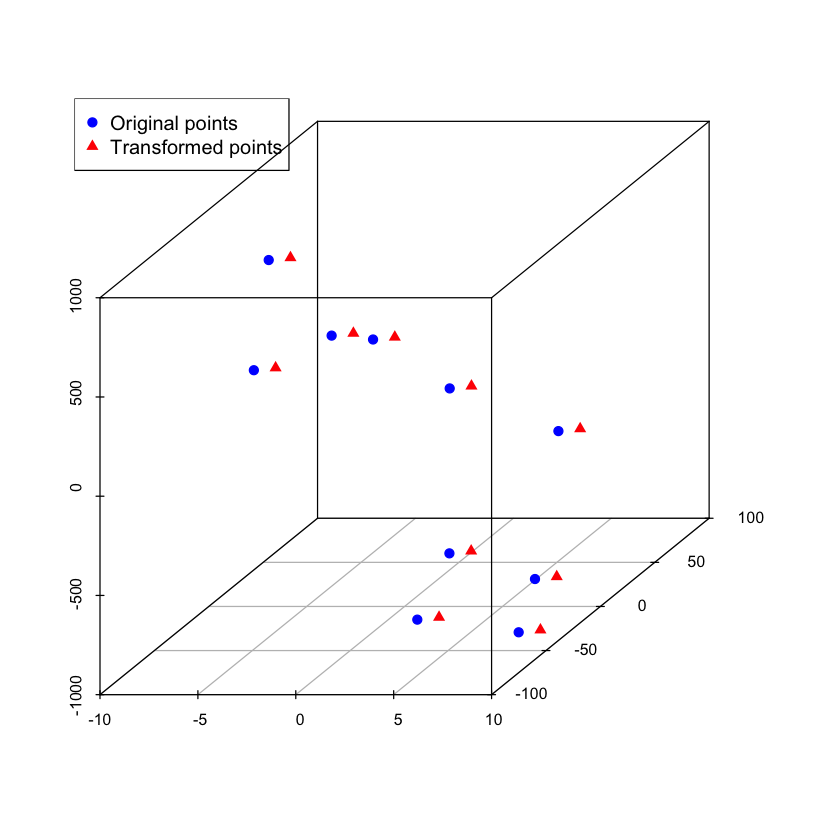## Rotation to Rigid [3D]¶ Copy the matrix or versor and center of rotation. In : rotationCenter <- c(10, 10, 10) rotation <- VersorTransform(c(0,0,1,0), rotationCenter) rigid_euler <- Euler3DTransform() rigid_euler$SetMatrix(rotation$GetMatrix()) rigid_euler$SetCenter(rotation$GetCenter()) rigid_versor <- VersorRigid3DTransform() rigid_versor$SetRotation(rotation$GetVersor()) #rigid_versor.SetCenter(rotation.GetCenter()) #intentional error # Sanity check to make sure the transformations are equivalent. bounds <- list(c(-10,10),c(-100,100), c(-1000,1000)) num_points = 10 point_data = uniform_random_points(bounds, num_points) transformed_point_data <- apply(point_data, MARGIN=2, rotation$TransformPoint)

euler_errors = target_registration_errors(rigid_euler, point_data, transformed_point_data)
versor_errors = target_registration_errors(rigid_versor, point_data, transformed_point_data)

# Draw the points transformed by the original transformation and after transformation
# using the incorrect transformation, illustrate the effect of center of rotation.
incorrect_transformed_point_data <- apply(point_data, 2, rigid_versor$TransformPoint) all_data <- cbind(transformed_point_data, incorrect_transformed_point_data) xbnd <- range(all_data[1,]) ybnd <- range(all_data[2,]) zbnd <- range(all_data[3,]) s3d <- scatterplot3d(t(transformed_point_data), color = "blue", pch = 19, xlab='', ylab='', zlab='', xlim=xbnd, ylim=ybnd, zlim=zbnd) s3d$points3d(t(incorrect_transformed_point_data), col = "red", pch = 17)
legend("topleft", col= c("blue", "red"), pch=c(19,17), legend = c("Original points", "Transformed points"))

cat(paste0("Euler\tminError:", point2str(min(euler_errors))," maxError: ", point2str(max(euler_errors)),"\n"))
cat(paste0("Versor\tminError:", point2str(min(versor_errors))," maxError: ", point2str(max(versor_errors)),"\n"))

Euler	minError:0.0 maxError: 0.0
Versor	minError:28.3 maxError: 28.3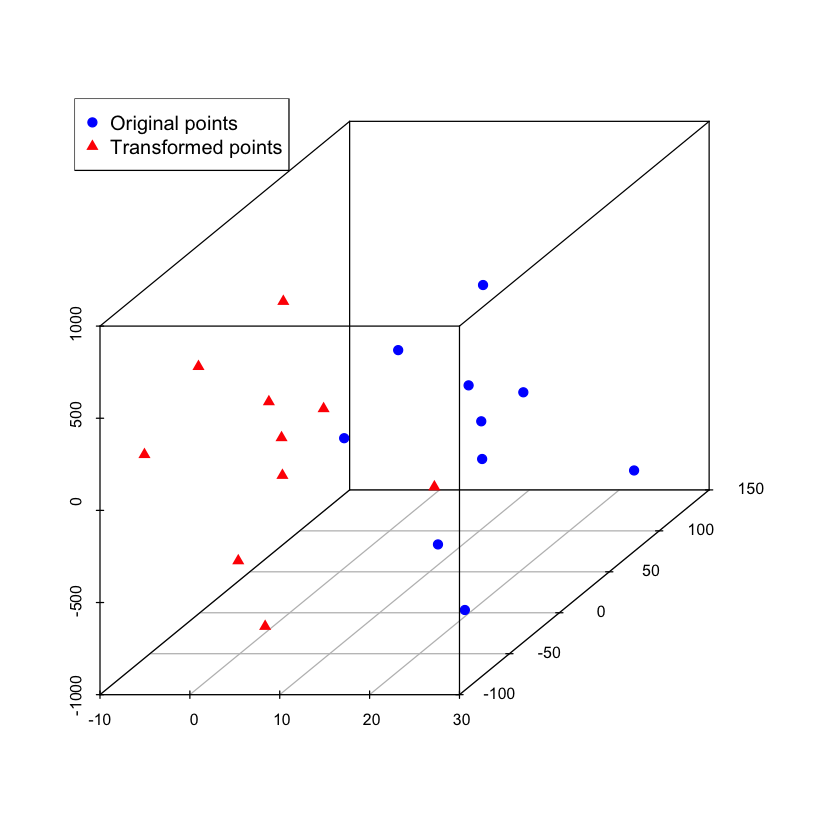## Similarity [2D]¶

When the center of the similarity transformation is not at the origin the effect of the transformation is not what most of us expect. This is readily visible if we limit the transformation to scaling: $T(\mathbf{x}) = s\mathbf{x}-s\mathbf{c} + \mathbf{c}$. Changing the transformation's center results in scale + translation.

In :
# 2D square centered on (0,0)
points <- matrix(data=c(-1.0,-1.0, -1.0,1.0, 1.0,1.0, 1.0,-1.0), ncol=4, nrow=2)
# Scale by 2 (center default is [0,0])
similarity <- Similarity2DTransform();
similarity$SetScale(2) scaled_points <- apply(points, MARGIN=2, similarity$TransformPoint)

#Uncomment the following lines to change the transformations center and see what happens:
#similarity$SetCenter(c(0,2)) #scaled_points <- apply(points, 2, similarity$TransformPoint)

plot(points[1,],points[2,], xlim=c(-10,10), ylim=c(-10,10), pch=19, col="blue", xlab="", ylab="", las=1)
points(scaled_points[1,], scaled_points[2,], col="red", pch=17)
legend('top', col= c("red", "blue"), pch=c(17,19), legend = c("transformed points", "original points"))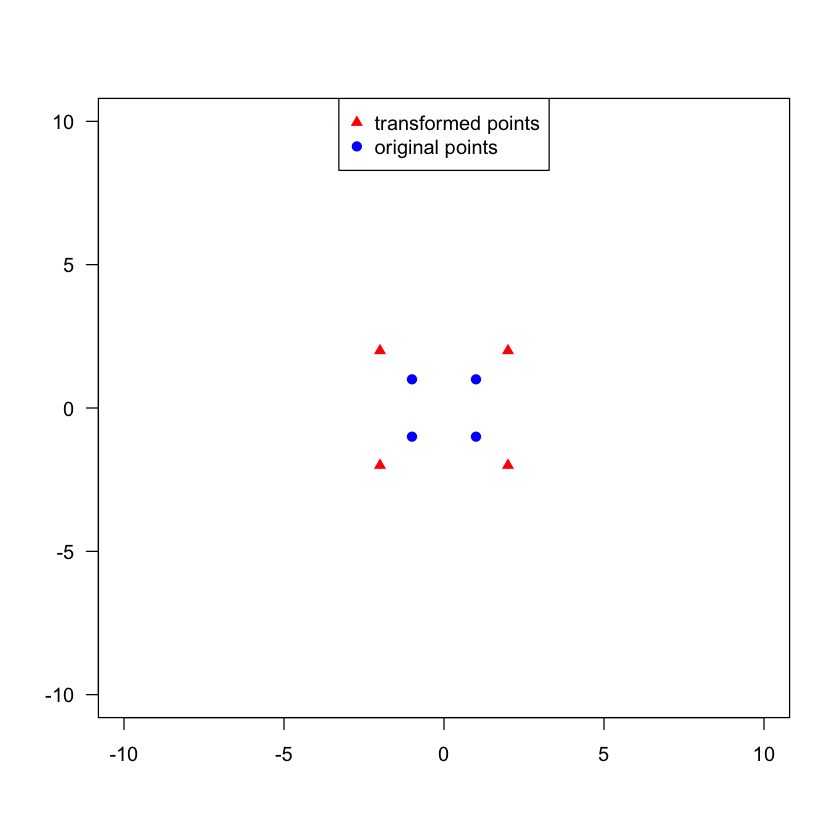## Rigid to Similarity [3D]¶

Copy the translation, center, and matrix or versor.

In :
rotation_center <- c(100, 100, 100)
theta_x <- 0.0
theta_y <- 0.0
theta_z <- pi/2.0
translation <- c(1,2,3)

rigid_euler <- Euler3DTransform(rotation_center, theta_x, theta_y, theta_z, translation)

similarity <- Similarity3DTransform()
similarity$SetMatrix(rigid_euler$GetMatrix())
similarity$SetTranslation(rigid_euler$GetTranslation())
similarity$SetCenter(rigid_euler$GetCenter())

# Apply the transformations to the same set of random points and compare the results
# (see utility functions at top of notebook).
print_transformation_differences(rigid_euler, similarity)

Euler3DTransform - Similarity3DTransform :	minDifference:  0  maxDifference:  0

## Similarity to Affine [3D]¶

Copy the translation, center and matrix.

In :
rotation_center <- c(100, 100, 100)
axis <- c(0,0,1)
angle <- pi/2.0
translation <- c(1,2,3)
scale_factor <- 2.0
similarity <- Similarity3DTransform(scale_factor, axis, angle, translation, rotation_center)

affine <- AffineTransform(3)
affine$SetMatrix(similarity$GetMatrix())
affine$SetTranslation(similarity$GetTranslation())
affine$SetCenter(similarity$GetCenter())

# Apply the transformations to the same set of random points and compare the results
# (see utility functions at top of notebook).
print_transformation_differences(similarity, affine)

Similarity3DTransform - AffineTransform :	minDifference:  0  maxDifference:  0

## Scale Transform¶

Just as the case was for the similarity transformation above, when the transformations center is not at the origin, instead of a pure anisotropic scaling we also have translation ($T(\mathbf{x}) = \mathbf{s}^T\mathbf{x}-\mathbf{s}^T\mathbf{c} + \mathbf{c}$).

In :
# 2D square centered on (0,0).
points <- matrix(data=c(-1.0,-1.0, -1.0,1.0, 1.0,1.0, 1.0,-1.0), ncol=4, nrow=2)

# Scale by half in x and 2 in y.
scale <- ScaleTransform(2, c(0.5,2));

scaled_points <- apply(points, 2, scale$TransformPoint) #Uncomment the following lines to change the transformations center and see what happens: #scale$SetCenter(c(0,2))
#scaled_points <- apply(points, 2, scale$TransformPoint) plot(points[1,],points[2,], xlim=c(-10,10), ylim=c(-10,10), pch=19, col="blue", xlab="", ylab="", las=1) points(scaled_points[1,], scaled_points[2,], col="red", pch=17) legend('top', col= c("red", "blue"), pch=c(17,19), legend = c("transformed points", "original points"))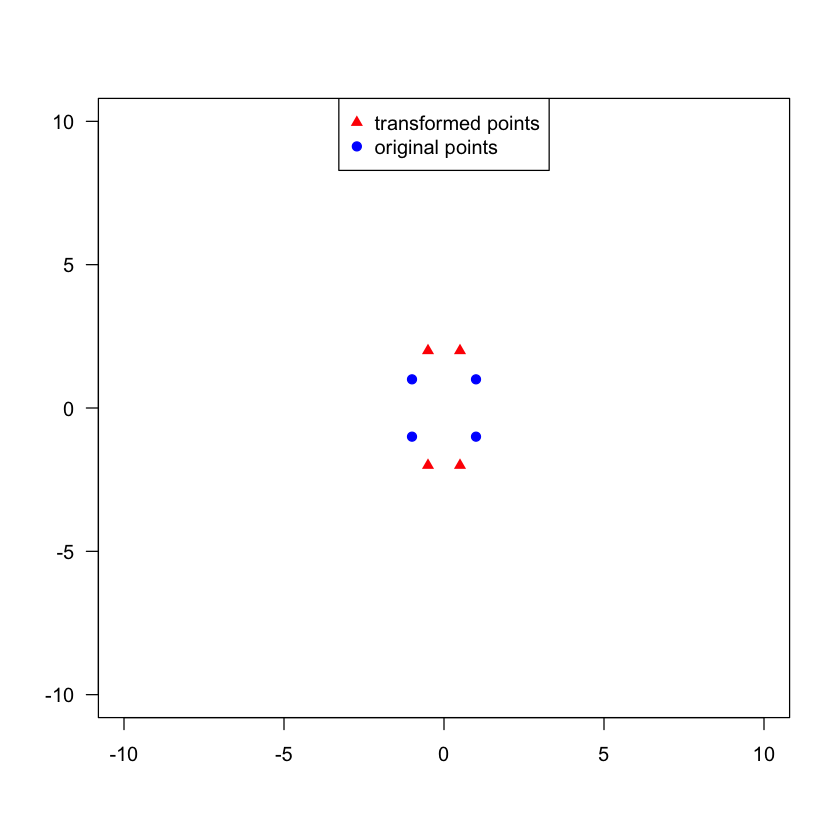## Scale Versor¶ This is not what you would expect from the name (composition of anisotropic scaling and rigid). This is: $$T(x) = (R+S)(\mathbf{x}-\mathbf{c}) + \mathbf{t} + \mathbf{c},\;\; \textrm{where } S= \left[\begin{array}{ccc} s_0-1 & 0 & 0 \\ 0 & s_1-1 & 0 \\ 0 & 0 & s_2-1 \end{array}\right]$$ There is no natural way of "promoting" the similarity transformation to this transformation. In : scales <- c(0.5,0.7,0.9) translation <- c(1,2,3) axis <- c(0,0,1) angle <- 0.0 scale_versor <- ScaleVersor3DTransform(scales, axis, angle, translation) print(scale_versor)  itk::simple::ScaleVersor3DTransform ScaleVersor3DTransform (0x7f9c2dde33e0) RTTI typeinfo: itk::ScaleVersor3DTransform<double> Reference Count: 1 Modified Time: 853 Debug: Off Object Name: Observers: none Matrix: 0.5 0 0 0 0.7 0 0 0 0.9 Offset: [1, 2, 3] Center: [0, 0, 0] Translation: [1, 2, 3] Inverse: 2 0 0 0 1.42857 0 0 0 1.11111 Singular: 0 Versor: [ 0, 0, 0, 1 ] Scales: [0.5, 0.7, 0.9]  ## Scale Skew Versor¶ Again, not what you expect based on the name, this is not a composition of transformations. This is: $$T(x) = (R+S+K)(\mathbf{x}-\mathbf{c}) + \mathbf{t} + \mathbf{c},\;\; \textrm{where } S = \left[\begin{array}{ccc} s_0-1 & 0 & 0 \\ 0 & s_1-1 & 0 \\ 0 & 0 & s_2-1 \end{array}\right]\;\; \textrm{and } K = \left[\begin{array}{ccc} 0 & k_0 & k_1 \\ k_2 & 0 & k_3 \\ k_4 & k_5 & 0 \end{array}\right]$$ In practice this is an over-parametrized version of the affine transform, 15 (scale, skew, versor, translation) vs. 12 parameters (matrix, translation). In : scale <- c(2,2.1,3) skew <- 0:1/6.0:1 #six equally spaced values in[0,1], an arbitrary choice translation <- c(1,2,3) versor <- c(0,0,0,1.0) scale_skew_versor <- ScaleSkewVersor3DTransform(scale, skew, versor, translation) print(scale_skew_versor)  itk::simple::ScaleSkewVersor3DTransform ScaleSkewVersor3DTransform (0x7f9c3282ae70) RTTI typeinfo: itk::ScaleSkewVersor3DTransform<double> Reference Count: 1 Modified Time: 863 Debug: Off Object Name: Observers: none Matrix: 2 0 0.2 0 2.1 0.333333 0 1 3 Offset: [1, 2, 3] Center: [0, 0, 0] Translation: [1, 2, 3] Inverse: 0.5 0.0167598 -0.0351955 2.77556e-17 0.502793 -0.0558659 -9.71445e-17 -0.167598 0.351955 Singular: 0 Versor: [ 0, 0, 0, 1 ] Scale: [2, 2.1, 3] Skew: [0, 0.2, 0, 0.333333, 0, 1]  ## Bounded Transformations¶ SimpleITK supports two types of bounded non-rigid transformations, BSplineTransform (sparse representation) and DisplacementFieldTransform (dense representation). Transforming a point that is outside the bounds will return the original point - identity transform. In : # # This function displays the effects of the deformable transformation on a grid of points by scaling the # initial displacements (either of control points for BSpline or the deformation field itself). It does # assume that all points are contained in the range(-2.5,-2.5), (2.5,2.5) - for display. # display_displacement_scaling_effect <- function(s, original_x_mat, original_y_mat, tx, original_control_point_displacements) { if(tx$GetDimension()!=2)
stop('display_displacement_scaling_effect only works in 2D')

tx$SetParameters(s*original_control_point_displacements) transformed_points <- mapply(function(x,y) tx$TransformPoint(c(x,y)), original_x_mat, original_y_mat)

plot(original_x_mat,original_y_mat, xlim=c(-2.5,2.5), ylim=c(-2.5,2.5), pch=19, col="blue", xlab="", ylab="", las=1)
points(transformed_points[1,], transformed_points[2,], col="red", pch=17)
legend('top', col= c("red", "blue"), pch=c(17,19), legend = c("transformed points", "original points"))
}


## BSpline¶

Using a sparse set of control points to control a free form deformation.

In :
# Create the transformation (when working with images it is easier to use the BSplineTransformInitializer function
# or its object oriented counterpart BSplineTransformInitializerFilter).
dimension <- 2
spline_order <- 3
direction_matrix_row_major <- c(1.0,0.0,0.0,1.0) # identity, mesh is axis aligned
origin <- c(-1.0,-1.0)
domain_physical_dimensions <- c(2,2)

bspline <- BSplineTransform(dimension, spline_order)
bspline$SetTransformDomainOrigin(origin) bspline$SetTransformDomainDirection(direction_matrix_row_major)
bspline$SetTransformDomainPhysicalDimensions(domain_physical_dimensions) bspline$SetTransformDomainMeshSize(c(4,3))

# Random displacement of the control points.
originalControlPointDisplacements <- runif(length(bspline$GetParameters())) bspline$SetParameters(originalControlPointDisplacements)

# Apply the bspline transformation to a grid of points
# starting the point set exactly at the origin of the bspline mesh is problematic as
# these points are considered outside the transformation's domain,
# remove epsilon below and see what happens.
numSamplesX = 10
numSamplesY = 20

eps <- .Machine$double.eps coordsX <- seq(origin + eps, origin + domain_physical_dimensions, (domain_physical_dimensions-eps)/(numSamplesX-1)) coordsY <- seq(origin + eps, origin + domain_physical_dimensions, (domain_physical_dimensions-eps)/(numSamplesY-1)) # next two lines equivalent to Python's/MATLAB's meshgrid XX <- outer(coordsY*0, coordsX, "+") YY <- outer(coordsY, coordsX*0, "+") display_displacement_scaling_effect(0.0, XX, YY, bspline, originalControlPointDisplacements) #uncomment the following line to see the effect of scaling the control point displacements # on our set of points (we recommend keeping the scaling in the range [-1.5,1.5] due to display bounds) #display_displacement_scaling_effect(0.5, XX, YY, bspline, originalControlPointDisplacements)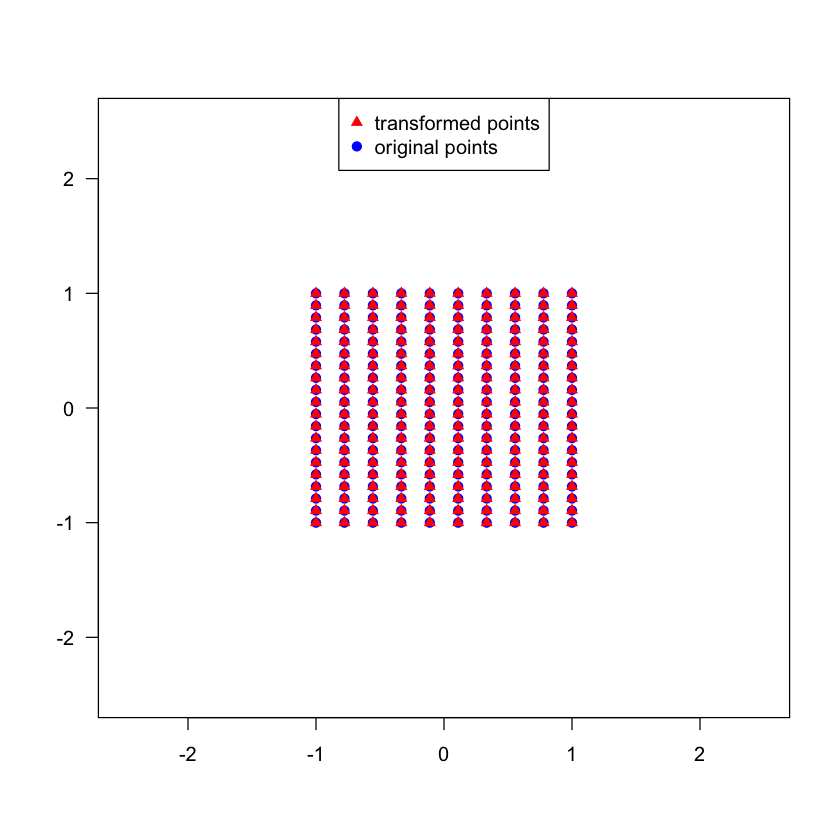## DisplacementField¶ A dense set of vectors representing the displacement inside the given domain. The most generic representation of a transformation. In : # Create the displacement field. # When working with images the safer thing to do is use the image based constructor, # DisplacementFieldTransform(my_image), all the fixed parameters will be set correctly and the displacement # field is initialized using the vectors stored in the image. SimpleITK requires that the image's pixel type be # "sitkVectorFloat64". displacement <- DisplacementFieldTransform(2) field_size <- c(10,20) field_origin <- c(-1.0,-1.0) field_spacing <- c(2.0/9.0,2.0/19.0) field_direction <- c(1,0,0,1) # direction cosine matrix (row major order) # Concatenate all the information into a single list displacement$SetFixedParameters(c(field_size, field_origin, field_spacing, field_direction))
# Set the interpolator, either sitkLinear which is default or nearest neighbor
displacement$SetInterpolator("sitkNearestNeighbor") originalDisplacements <- runif(length(displacement$GetParameters()))
displacement$SetParameters(originalDisplacements) coordsX <- seq(field_origin, field_origin+(field_size-1)*field_spacing, field_spacing) coordsY <- seq(field_origin, field_origin+(field_size-1)*field_spacing, field_spacing) # next two lines equivalent to Python's/MATLAB's meshgrid XX <- outer(coordsY*0, coordsX, "+") YY <- outer(coordsY, coordsX*0, "+") display_displacement_scaling_effect(0.0, XX, YY, displacement, originalDisplacements) #uncomment the following line to see the effect of scaling the control point displacements # on our set of points (we recommend keeping the scaling in the range [-1.5,1.5] due to display bounds) #display_displacement_scaling_effect(0.5, XX, YY, displacement, originalDisplacements)Displacement field transform created from an image. Remember that SimpleITK will clear the image you provide, as shown in the cell below. In : displacement_image <- Image(c(64,64), "sitkVectorFloat64") # The only point that has any displacement is at physical SimpleITK index (0,0), R index (1,1) displacement <- c(0.5,0.5) # Note that SimpleITK indexing starts at zero. displacement_image$SetPixel(c(0,0), displacement)

cat('Original displacement image size: ',point2str(displacement_image$GetSize()),"\n") displacement_field_transform <- DisplacementFieldTransform(displacement_image) cat("After using the image to create a transform, displacement image size: ", point2str(displacement_image$GetSize()), "\n")

# Check that the displacement field transform does what we expect.
cat("Expected result: ",point2str(displacement),
"\nActual result: ", displacement_field_transform$TransformPoint(c(0,0)),"\n")  Original displacement image size: 64.0, 64.0 After using the image to create a transform, displacement image size: 0.0, 0.0 Expected result: 0.5, 0.5 Actual result: 0.5 0.5  ## Composite transform (Transform)¶ The generic SimpleITK transform class. This class can represent both a single transformation (global, local), or a composite transformation (multiple transformations applied one after the other). This is the output typed returned by the SimpleITK registration framework. The choice of whether to use a composite transformation or compose transformations on your own has subtle differences in the registration framework. Below we represent the composite transformation$T_{affine}(T_{rigid}(x))$in two ways: (1) use a composite transformation to contain the two; (2) combine the two into a single affine transformation. We can use both as initial transforms (SetInitialTransform) for the registration framework (ImageRegistrationMethod). The difference is that in the former case the optimized parameters belong to the rigid transformation and in the later they belong to the combined-affine transformation. In : # Create a composite transformation: T_affine(T_rigid(x)). rigid_center <- c(100,100,100) theta_x <- 0.0 theta_y <- 0.0 theta_z <- pi/2.0 rigid_translation <- c(1,2,3) rigid_euler <- Euler3DTransform(rigid_center, theta_x, theta_y, theta_z, rigid_translation) affine_center <- c(20, 20, 20) affine_translation <- c(5,6,7) # Matrix is represented as a vector-like data in row major order. affine_matrix <- runif(9) affine <- AffineTransform(affine_matrix, affine_translation, affine_center) # Using the composite transformation we just add them in (stack based, first in - last applied). composite_transform <- Transform(affine) composite_transform$AddTransform(rigid_euler)

# Create a single transform manually. this is a recipe for compositing any two global transformations
# into an affine transformation, T_0(T_1(x)):
# A = A=A0*A1
# c = c1
# t = A0*[t1+c1-c0] + t0+c0-c1
A0 <- t(matrix(affine$GetMatrix(), 3, 3)) c0 <- affine$GetCenter()
t0 <- affine$GetTranslation() A1 <- t(matrix(rigid_euler$GetMatrix(), 3, 3))
c1 <- rigid_euler$GetCenter() t1 <- rigid_euler$GetTranslation()

combined_mat <- A0%*%A1
combined_center <- c1
combined_translation <- A0 %*% (t1+c1-c0) + t0+c0-c1
combined_affine <- AffineTransform(c(t(combined_mat)), combined_translation, combined_center)

# Check if the two transformations are "equivalent".
cat("Apply the two transformations to the same point cloud:\n")
print_transformation_differences(composite_transform, combined_affine)

cat("\nTransform parameters:\n")
cat(paste("\tComposite transform: ", point2str(composite_transform$GetParameters(),2),"\n")) cat(paste("\tCombined affine: ", point2str(combined_affine$GetParameters(),2),"\n"))

cat("Fixed parameters:\n")
cat(paste("\tComposite transform: ", point2str(composite_transform$GetFixedParameters(),2),"\n")) cat(paste("\tCombined affine: ", point2str(combined_affine$GetFixedParameters(),2),"\n"))

Apply the two transformations to the same point cloud:
Transform - AffineTransform :	minDifference:  0  maxDifference:  6.35528743231302e-14
Transform parameters:
Composite transform:  0.00, 0.00, 1.57, 1.00, 2.00, 3.00
Combined affine:  0.74, -0.96, 0.20, 0.80, -0.54, 0.79, 0.20, -0.16, 0.48, 80.74, 100.16, -4.00
Fixed parameters:
Composite transform:  100.00, 100.00, 100.00, 0.00
Combined affine:  100.00, 100.00, 100.00


Composite transforms enable a combination of a global transformation with multiple local/bounded transformations. This is useful if we want to apply deformations only in regions that deform while other regions are only effected by the global transformation.

The following code illustrates this, where the whole region is translated and subregions have different deformations.

In :
# Global transformation.
translation <- TranslationTransform(2, c(1.0,0.0))

# Displacement in region 1.
displacement1 <- DisplacementFieldTransform(2)
field_size <- c(10,20)
field_origin <- c(-1.0,-1.0)
field_spacing <- c(2.0/9.0,2.0/19.0)
field_direction <- c(1,0,0,1) # direction cosine matrix (row major order)

# Concatenate all the information into  a single list.
displacement1$SetFixedParameters(c(field_size, field_origin, field_spacing, field_direction)) displacement1$SetParameters(rep(1.0, length(displacement1$GetParameters()))) # Displacement in region 2. displacement2 <- DisplacementFieldTransform(2) field_size <- c(10,20) field_origin <- c(1.0,-3) field_spacing <- c(2.0/9.0,2.0/19.0) field_direction <- c(1,0,0,1) #direction cosine matrix (row major order) # Concatenate all the information into a single list. displacement2$SetFixedParameters(c(field_size, field_origin, field_spacing, field_direction))
displacement2$SetParameters(rep(-1.0, length(displacement2$GetParameters())))

# Composite transform which applies the global and local transformations.
composite <- Transform(translation)
composite$AddTransform(displacement1) composite$AddTransform(displacement2)

# Apply the composite transformation to points in ([-1,-3],[3,1]) and
# display the deformation using a quiver plot.

# Generate points.
numSamplesX <- 10
numSamplesY <- 10
coordsX <- seq(-1.0, 3.0, 4.0/(numSamplesX-1))
coordsY <- seq(-3.0, 1.0, 4.0/(numSamplesY-1))
# next two lines equivalent to Python's/MATLAB's meshgrid
original_x_mat <- outer(coordsY*0, coordsX, "+")
original_y_mat <- outer(coordsY, coordsX*0, "+")

# Transform points and plot.
original_points <- mapply(function(x,y) c(x,y), original_x_mat, original_y_mat)
transformed_points <- mapply(function(x,y) composite$TransformPoint(c(x,y)), original_x_mat, original_y_mat) plot(0,0,xlim=c(-1.0,3.0), ylim=c(-3.0,1.0), las=1) arrows(original_points[1,], original_points[2,], transformed_points[1,], transformed_points[2,])## Writing and Reading¶ The SimpleITK.ReadTransform() returns a SimpleITK.Transform . The content of the file can be any of the SimpleITK transformations or a composite (set of transformations). In : # Create a 2D rigid transformation, write it to disk and read it back. basic_transform <- Euler2DTransform() basic_transform$SetTranslation(c(1,2))
basic_transform$SetAngle(pi/2.0) full_file_name <- file.path(OUTPUT_DIR, "euler2D.tfm") WriteTransform(basic_transform, full_file_name) # The ReadTransform function returns a SimpleITK Transform no matter the type of the transform # found in the file (global, bounded, composite). read_result <- ReadTransform(full_file_name) cat(paste("Original type: ",basic_transform$GetName(),"\nType after reading: ", read_result$GetName(),"\n")) print_transformation_differences(basic_transform, read_result) # Create a composite transform then write and read. displacement <- DisplacementFieldTransform(2) field_size <- c(10,20) field_origin <- c(-10.0,-100.0) field_spacing <- c(20.0/(field_size-1),200.0/(field_size-1)) field_direction <- c(1,0,0,1) #direction cosine matrix (row major order) # Concatenate all the information into a single list. displacement$SetFixedParameters(c(field_size, field_origin, field_spacing, field_direction))
displacement$SetParameters(runif(length(displacement$GetParameters())))

composite_transform <- Transform(basic_transform)

Original type:  Euler2DTransform
Transform - Transform :	minDifference:  0  maxDifference:  0